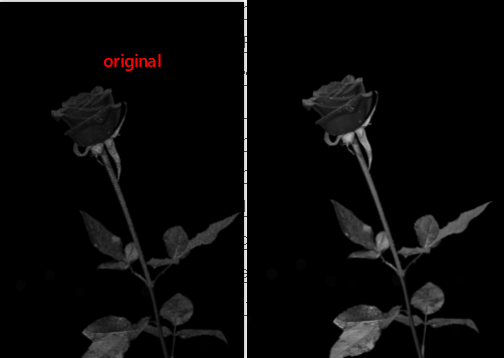# Python OpenCV: Remove Noise in Image Using Contraharmonic Mean Filter

In this tutorial, we will introduce how to remove image noise using contraharmonic mean filter in python opencv.1.Open an image with noise

```import numpy as np
import cv2

2.Generate contraharmonic mean filter kernel

```def contraharmonic_mean(img, size, Q):
num = np.power(img, Q + 1)
denom = np.power(img, Q)
kernel = np.full(size, 1.0)
result = cv2.filter2D(num, -1, kernel) / cv2.filter2D(denom, -1, kernel)
return result```

3.Implement contraharmonic  mean filter to remove noise in image

`cv2.imshow(contraharmonic_mean(img_src, (3,3), 0.5))`

Run this code, you may get this image: